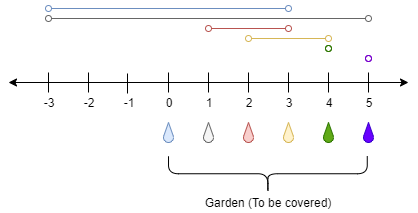# 灌溉花园的最少水龙头数目

## 1326. 灌溉花园的最少水龙头数目 (Hard)```输入：n = 5, ranges = [3,4,1,1,0,0]

```

```输入：n = 3, ranges = [0,0,0,0]

```

```输入：n = 7, ranges = [1,2,1,0,2,1,0,1]

```

```输入：n = 8, ranges = [4,0,0,0,0,0,0,0,4]

```

```输入：n = 8, ranges = [4,0,0,0,4,0,0,0,4]

```

• `1 <= n <= 10^4`
• `ranges.length == n + 1`
• `0 <= ranges[i] <= 100`

[贪心算法] [动态规划]